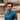March 25, 2020

# What is S.O.L.I.D by the way?

In object-oriented computer programming, SOLID is a mnemonic acronym for five design principles intended to make software designs more understandable, flexible and maintainable.

SOLID is an acronym for 5 important design principles when doing OOP. It was first introduced by Robert C. Martin (Uncle Bob), in his 2000 paper Design Principles and Design Patterns.

SOLID stands for -

• S - Single Responsibility Principle
• O - Open/Closed Principle
• L - Liskov’s Substitution Principle
• I - Implementation Segregation Principle
• D - Dependency Inversion Principle

In this article, I will be covering O - Open/Closed Principle. Note - The examples will be in Java, but applies to any OOP language.

# O - Open/Closed Principle (OCP)

The Open/Closed Principle (OCP) is the SOLID principle which states

Software entities (classes, modules, functions, etc.) should be open for extension but closed for modification.

In simple words, we should strive to write code that can be extended without the need for modification, when a change request is made by the customer or a product owner.

# Anti OCP usage

We will first look at an example that breaks the OCP principle.

``````// GeneralInvestment.java
public class GeneralInvestment {
public Double initialAmount;
public int year;
public Double tax;

public GeneralInvestment(Double initialAmount, int year, Double tax) {
this.initialAmount = initialAmount;
this.year = year;
this.tax = tax;
}
}

//InvestmentManager.java
public final class InvestmentManager {
public Double calculateReturn(GeneralInvestment investment) {
return investment.initialAmount + (investment.year * investment.initialAmount * investment.tax);
}
}``````

So, we have a `GeneralInvestment` class and an `InvestmentManager` class. The sole purpose of `InvestmentManager` is to calculate return of type `GeneralInvestment`.

Going further we have a feature request to calculate return on `AdvancedInvestment`. Let’s add some code to accomplish that.

``````//AdvancedInvestment.java
public class AdvancedInvestment extends GeneralInvestment {
public Double incremental;
public int period;

public AdvancedInvestment(Double initialAmount, int year, Double tax, Double incremental, int period) {
super(initialAmount, year, tax);
this.incremental = incremental;
this.period = period;
}
}``````

Now, we have `AdvancedInvestment` in place. Let’s update our `InvestmentManager` class to handle new case.

``````public final class InvestmentManager {
public Double calculatereturn(GeneralInvestment investment) {
} else {
return investment.initialAmount + (investment.year * investment.initialAmount * investment.tax);
}
}
}``````

This works! But, it violates the OCP since `InvestmentManager` class is

• Closed for extension
• Open for modification

# OCP in action

Let’s try and implement the above-mentioned scenario without breaking OCP.

Step 1: Create an interface

``````// Investment.java
interface Investment {
public Double calculateReturn();
}``````

Step 2: Update `GeneralInvestment` and `AdvancedInvestment` classes by implementing `Investment` interface

``````//GeneralInvestment.java
public class GeneralInvestment implements Investment {
public Double initialAmount;
public int year;
public Double tax;

public GeneralInvestment(Double initialAmount, int year, Double tax) {
this.initialAmount = initialAmount;
this.year = year;
this.tax = tax;
}

public Double calculateReturn() {
return initialAmount + (year * initialAmount * tax);
}
}

public class AdvancedInvestment implements Investment {
public Double incremental;
public int period;
public GeneralInvestment investment;

public AdvancedInvestment(Double initialAmount, int year, Double tax, Double incremental, int period) {
this.incremental = incremental;
this.period = period;
this.investment = new GeneralInvestment(initialAmount, year, tax);
}

public Double calculateReturn() {
return investment.initialAmount + (investment.year * investment.initialAmount * investment.tax)
+ (period * incremental * investment.tax);
}
}``````

Step 3: FInally update the `InvestmentManager` class

``````//InvestmentManager.java
public final class InvestmentManager {
public Double calculateReturn(Investment investment) {
return investment.calculateReturn();
}
}``````

Here is the UML diagram to reduce verbosity:Here we go! Going further if we have a new requirement to add different types of Investment we can create a new class, implement the `Investment` interface and we are done. No need to modify any existing code.

# TL;DR

Always keep in mind to make your code open for extension and closed for modification. This will make the maintenance of code so easy.

The example in this post has used a compositional design pattern (Strategy Pattern) to achieve OCP, but it can also be achieved through the use of inheritance.Personal thought by Abhishek Prakash
A platform to share my thoughts